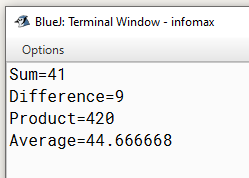# Java program to average of 36,45 and 53 using variables of adequate data type.

Write a program to average of 36,45 and 53 using variables of adequate data type.

`Solution`

``````class Demo
{
public static void main(String arr[])
{
int a=36,b=45,c=53;
float d;
d=(float)(a+b+c)/3;
System.out.println("Average="+d);
}
}
``````
`Output`